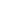Ebook

#### How to Weight Survey DataWeighting (also known as sample balancing, post-survey adjustment, raking, and poststratification) is the technique of adjusting survey results to bring them into line with some known characteristics of the population. For example, if a sample contains 40% males and the population contains 49% males, weighting can be used to adjust for this discrepancy.

#### What you'll get from this ebook

This How To Weight Survey Data ebook describes all the key steps involved in weighting survey data (also known as sample balancing).

• Raking
• Poststratification
• Non-response weighting

Commercial weighting tends to be different to that used in academic studies which is in-turn, different to that used in official statistics and other government statistics. This book focuses on the commercial applications of weighting.1

A key aspect of commercial weighting is that typically one or a small number of weight variables are created for use in many subsequent analyses. Where there is only a single analysis of primary interest, such as a single regression model or an opinion poll, alternative approaches may be preferable, including:

• Performing a regression with the adjustment variables that would have been used to create the weights (see the next chapter) included as predictors.
• Using MrP (“Mister P”). See: http://www.misterp.org/papers.html.

Using market share as a numeric adjustment variable
Using dates (waves) in composite variables
The weighting variable(s)

Creating sampling weights
Design weights
Cell weights
Rim weights/Raking
Calibration weights
Calibrated weight
Volumetric weights
Weights for different purposes
Combining weights
More exotic type of weights
Frequency weights
Variance weights
Replicate weights
Replication weights
Expansion weights
Analytic weights
Importance weights
Self-weighting samples

Common mistakes
No sample for a target group
Categorical targets that add up to 0.99999 or 1.00001
Impossible numeric targets
Inconsistent categorical targets
Inconsistent numeric targets
Ignoring measurement error
Using weight factors instead of targets

Checking weights
Case study
The average of the weight variable
The range of the weight variable
The distribution of the weight variable
Effective sample size

Improving a weight
Changing the targets
Trimming (bounds on weights)

Analysis of weighted data
Bias and statistical inference
Approaches to incorporating weights in statistical analyses
Variance estimation
Resampling
Weight calibration
Using the observed sample size
Modifying the weight to have an average weight of 1
Use the weight as is
Does choice of approach make a big difference? Yes
Approaches available by stat package
Q and Displayr
SPSS Statistics
R
When weighting does and does not work

Weighting in Displayr
Applying a weight to an analysis
Weighted versus unweighted data
Effective sample size
Using weights created in other software
Cell weighting with a single variable
Creating a composite categorical adjustment variable
More complex weights

Weighting in Q
Applying a weight to an analysis
Weighted versus unweighted data
Effective sample size
Using weights created in other software
Cell weights
Rim weights
More complex weights
Weighting in R
Applying a weight to an analysis
Creating weights